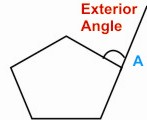HOME MATH DICTIONARY DOWNLOAD FEEDBACK DISCLAIMER
 Question: What is an Exterior Angle ? Answer: The Exterior Angle is the angle between any side of a shape, and a line extended from the next side. An exterior angle of a polygon is formed by extending one side of the polygon.When a side of a polygon is extended beyond the vertex, as at A, then the angle between the extended side and the adjacent side is called the exterior angle of the polygon at A. The sum of all the exterior angles of any polygon is 360°, as can be seen by an imaginary walk around the perimeter of the polygon and noticing the angles turned through.